Function Repository Resource:

# CollinearQ

Determine whether a set of points lie on a line

Contributed by: Wolfram|Alpha Math Team
 ResourceFunction["CollinearQ"][{list1,list2,…}] returns True if the set of points determined by the listi lie on a single line.

## Details and Options

ResourceFunction["CollinearQ"] accepts numeric and symbolic arguments.

## Examples

### Basic Examples (2)

Determine whether three points lie on a single line:

 In:=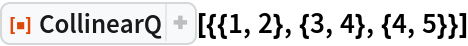Out=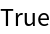In:=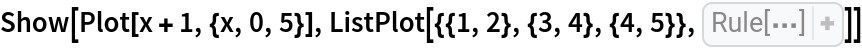Out=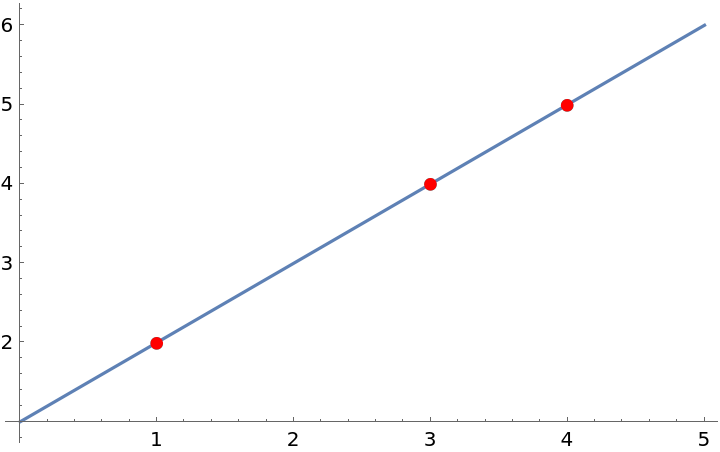A set of three points need not be colinear:

 In:=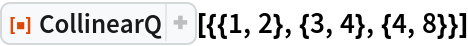Out=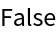In:=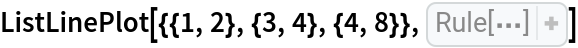Out=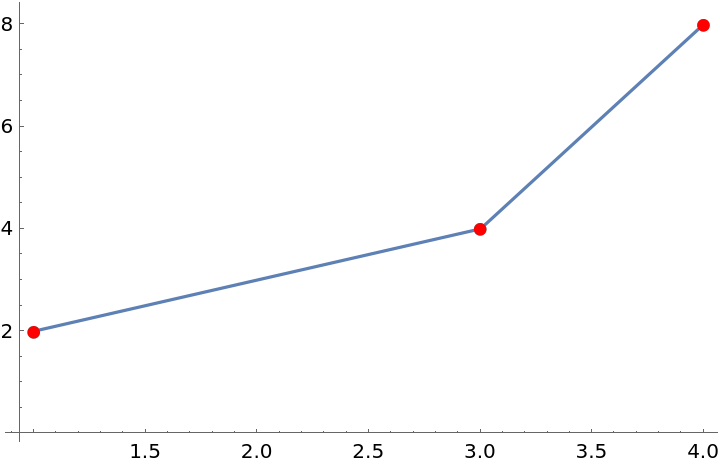### Scope (3)

CollinearQ works in more than two dimensions:

 In:=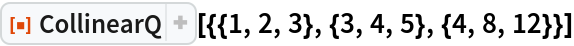Out=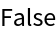Three points in three dimensions on a line:

 In:=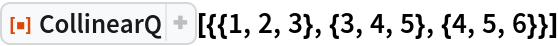Out=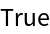CollinearQ works with symbolic input:

 In:=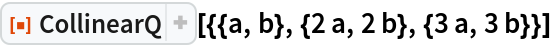Out=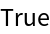## Publisher

Wolfram|Alpha Math Team

## Version History

• 2.0.0 – 23 March 2023
• 1.0.0 – 11 May 2020

## Author Notes

To view the code:

 In:=